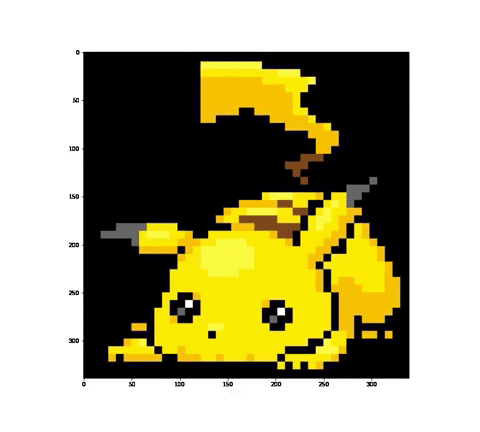DEV Community is a community of 787,688 amazing developers

We're a place where coders share, stay up-to-date and grow their careers.Animating two images transitioning horizontally with Python

Hi, I'll start with showing what I'm trying to achieve (I couldn't come up with a shorter title).We have two images, the second one slowly replaces the first (horizontally).

To do this we'll need to prepare two images with the same shape (I won't show how to scale them in python).

This method can be used to make any number of images transition (with a little bit of work).

First things first. Let's load two images.

# We'll need them later
import numpy as np
import matplotlib.pylab as plt

from PIL import Image

image1 = np.array(Image.open('image1.png').convert('RGB'))
image2 = np.array(Image.open('image2.png').convert('RGB'))

Both images are of shape: (339, 339, 3)

Generating frames

Firstly, let's flatten our images, we'll take advantage of that later.

fimage1 = image1.reshape((-1,3))
fimage2 = image2.reshape((-1,3))

To create animation we'll need to know how each frame will look. In my case I wanted to define a frame by a given part (perc) of pixels of the second image. For example if perc=0.1 then first 0.1 pixels are from second image, and 0.9 are from first. Fade is a function that will do that. Given two flattened images, the original shape of the image and perc, it will concatenate the two images and return the image to the original shape.

i = int(fimage1.shape * perc)
return np.concatenate((fimage2[:i], fimage1[i:, :])).reshape(shape)

Where:

• shape: tuple, shape of the original image
• fimage1: array, image flattened to shape (-1, 3)
• fimage2: same as fimage2
• perc: float, part of image2 to show

Here are some images that I generated with it:Creating animation

I won't go into details on how to create animations in Matplotlib, here's my code.

fig = plt.figure(figsize=(12, 10.8)) # Depends on aspect of your images
ax = plt.axes()
pic = ax.imshow(np.zeros(image1.shape)) # Create empty image of the same shape as image to plot
frames = 150 # Number of frames to generate

def init():
pic.set_array(np.zeros(image1.shape))
return [pic]

# This funtion generates i-th frame.
def animate(i):